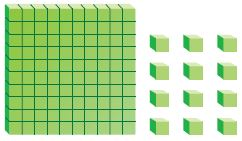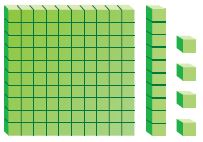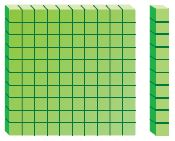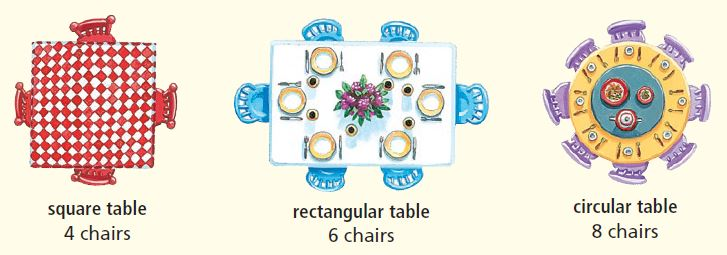# Go Math Grade 4 Answer Key Homework FL Chapter 4 Divide by 1-Digit Numbers Review/Test

Develop your math skills by referring to the Go Math Grade 4 Answer Key Homework FL Chapter 4 Divide by 1-Digit Numbers Review/Test. With the support of this HMH Go Math Grade 4 Review/Test Answer Key scholars can score good marks in their exam.

### Go Math Grade 4 Answer Key Homework FL Chapter 4 Divide by 1-Digit Numbers Review/Test

Go Math Grade 4 Answer Key Homework FL Review/Test covers all the topics in Chapter 4 Divide by 1-Digit Numbers. Explore the knowledge of your child by giving the question from Review/Test. Just hit on the link and download it. Go Math Grade 4 Answer Key Homework FL Chapter 4 Divide by 1-Digit Numbers Review/Test.

Chapter 4: Review/Test

### Review/Test – Page No. 187

Choose the best term from the box.Question 1.
1. When a number cannot be divided evenly, the amount left over is called the:

When a number cannot be divided evenly, the amount left over is called the remainder.

Question 2.
You use the _______________ method of dividing when multiples of the divisor are subtracted from the dividend and then the quotients are added together.

You use the compatible numbers method of dividing when multiples of the divisor are subtracted from the dividend and then the quotients are added together.

Use grid paper or base-ten blocks to model the quotient.

Then record the quotient.

Question 3.
96 ÷ 6 = ____

Explanation:

Question 4.
86 ÷ 2 = ____

Explanation:

Question 5.
155 ÷ 5 = ____

Explanation:

Find two numbers the quotient is between.
Then estimate the quotient.

Question 6.
787 ÷ 2
Estimate: ____

Explanation:
787 ÷ 2= 393.5
Estimate: 800 ÷ 2= 400.

Question 7.
391 ÷ 6
Estimate: ____

Explanation:
391 ÷ 6= 65.157
Estimate: 390 ÷ 6= 65

Question 8.
789 ÷ 8
Estimate: ____

Explanation:
789 ÷ 8= 98.62
Explanation: 800 ÷ 8= 100.

Divide.

Question 9.
3)$$\overline { 987 }$$
____

Explanation:
3)$$\overline { 987 }$$
= 987÷3
= 329.

Question 10.
7)$$\overline { 501 }$$
____ R ____

Explanation:
7)$$\overline { 501 }$$
= 501÷7
= 71 R 4.

Question 11.
5)$$\overline { 153 }$$
____ R ____

Explanation:
5)$$\overline { 153 }$$
= 153÷5
= 30 R 3.

Question 12.
4)$$\overline { 808 }$$
____ R ____

Explanation:
4)$$\overline { 808 }$$
= 808÷4
= 202 R 0.

Question 13.
6)$$\overline { 8,348 }$$
____ R ____

Explanation:
6)$$\overline { 8,348 }$$
= 8348÷6
= 1391 R 2.

Question 14.
8)$$\overline { 4,897 }$$
____ R ____

Explanation:
8)$$\overline { 4,897 }$$
= 4897÷8
= 612 R 1.

### Review/Test – Page No. 188

Question 15.
There are 96 tourists who have signed up to tour the island. The tourists are assigned to 6 equal-size groups. How many tourists are in each group?
Options:
a. 1 r3
b. 1 r6
c. 11
d. 16

Explanation:
As there are 96 tourists who have signed up to tour the island and the tourists are assigned to 6 equal-size groups. So the number of tourists are in each group is 96÷6= 16.

Question 16.
Maria needs to share the base-ten blocks equally among 4 equal groups.Which model shows how many are in each equal group?
Options:
a.b.c.d.Question 17.
Manny has 39 rocks. He wants to put the same number of rocks in each of 7 boxes. Which sentence shows how many rocks will be in each box?
Options:
a. He will need 6 boxes.
b. There will be 6 rocks in each box.
c. There will be 5 rocks in each box.
d. There will be 5 rocks left over.

Explanation:
As Manny has 39 rocks. He wants to put the same number of rocks in each of the 7 boxes, so there will be 5 rocks in each box.

### Review/Test – Page No. 189

Question 18.
There are 176 students in the marching band. They are arranged in equal rows of 8 students for a parade. How many rows of students are there?
Options:
a. 220 rows
b. 120 rows
c. 22 rows
d. 21 rows

Explanation:
As there are 176 students in the marching band and they arranged in equal rows of 8 students for a parade, so 176÷8= 22 rows of students are there.

Question 19.
Naomi wants to plant 387 tulip bulbs in 9 equal rows. She uses division to find the number of tulips in each row. In which place is the first digit of the quotient?
Options:
a. ones
b. tens
c. hundreds
d. thousands

Explanation:
Naomi wants to plant 387 tulip bulbs in 9 equal rows and she uses division to find the number of tulips in each row, so 387÷9= 43. And the first digit of the quotient is tens place.

Question 20.
Kevin and 2 friends are playing a game of cards. There are 52 cards in the deck to be shared equally. Kevin wants each player to receive the same number of cards. How many cards will each player receive? How many cards will be left over?
Options:
a. 16 cards and 4 cards left over
b. 17 cards and 1 card left over
c. 25 cards and 2 cards left over
d. 26 cards and no cards left over

Explanation:
Kevin and 2 friends are playing a game of cards and there are 52 cards in the deck to be shared equally, as Kevin wants each player to receive the same number of cards, each player will receive 52÷2= 26. So 26 cards each player receives and no cards left over.

Question 21.
Which number is the quotient?
1,125 ÷ 5 = ■
Options:
a. 25
b. 105
c. 125
d. 225

Explanation:
1,125 ÷ 5 =225.

### Review/Test – Page No. 190

Constructed Response

Question 22.
Mrs. Valdez bought 6 boxes of roses. Each box had 24 roses. She divided all the roses into 9 equal bunches. How many roses were in each bunch? Explain how to use a diagram to help solve the problem. Show your diagrams.
______ roses

Explanation:
As Mrs. Valdez bought 6 boxes of roses and each box had 24 roses, so the total number of roses are 6×24= 144 then she divided all the roses into 9 equal bunches. So each bunch will have 144÷9= 16 roses.

Question 23.
Mr. Owens plans to rent tables for a spaghetti fundraiser. He needs to seat 184 people.A. If Mr. Owens wants all rectangular tables, how many tables should he rent? Explain.
______ tables

Explanation:
The number of rectangular tables Mr. Owens should rent is 184÷6= 30.67. We will round off 30.67 to 31, so 31 tables he should rent.

Question 23.
B. Square tables rent for $12 each. Circular tables rent for$23 each. Mr. Owens says it would cost him less to rent square tables instead of circular tables. Is he right? Explain.

Answer: Yes, Mr. Owens is wrong.

Explanation:
As square tables rent for $12 each and circular tables rent for$23 each, so if Mr. Owens chooses square tables to rent and it has only 4 chairs, so 184÷4= 46 square tables should he rent which costs 46×$12=$552. And if Mr. Owens chooses circular tables he should rent 184÷8= 23 circular tables and which costs 23×$23=$529. So if he chooses circular tables he can pay less rent.

Conclusion:

The students of 4th grade can check all chapters in Go Math Grade Answer Key in pdf format so that your learning will kick start in a useful manner. We have given a brief explanation of each and every question on our Go Math Grade 4 Answer Key Homework FL Chapter 4 Divide by 1-Digit Numbers Review/Test. We suggest students to understand the concepts and apply them in the real world.

Scroll to Top